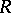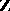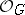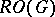# Bredon theory of modules over a category

(diff) ← Older revision | Latest revision (diff) | Newer revision → (diff)
A module over a small categoryis a contravariant functor fromto the category of-modules, for some commutative ring(cf. also Module). Taking morphisms to be natural transformations, the category of modules overis an Abelian category, so one can do homological algebra with these objects.
G.E. Bredon introduced modules over a category in [a1] in order to study obstruction theory for-spaces. Ifis a finite group, letbe the category of orbitsand-mappings between them.-modules (Abelian groups) overplay the role of the coefficients in Bredon's (-graded) equivariant ordinary cohomology theories. The equivariant ordinary cohomology of a-CW-complex can be computed using the equivariant chain complex of the space, which is a chain complex in the category of-modules over. Similarly, equivariant local cohomology can be described using modules over a category depending on the space in question.
For-graded equivariant ordinary cohomology one has to replace the orbit categorywith the stable orbit category, in which the morphisms are the stable mappings between orbits, stabilization being by all representations of. A module over the stable orbit category (i.e., an additive contravariant functor) is equivalent to a Mackey functor as studied by A.W.M. Dress in [a3].